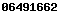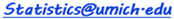# Bivariate Normal Distribution Interactive Calculator (3D)

Instructions
• Requirements - modern web-browser with HTML and JavaScript support. The 3D view of the bivariate distribution requires WebGL support.
• See the SOCR Bivariate Normal Distribution Activity.
• Use the Settings to initialize the web-app. To activate new input settings click outside the component.
• In the Control panel you can select the appropriate bivariate limits for the X and Y variables, choose desired Marginal or Conditional probability function, and view the 1D Normal Distribution graph. Use any non-numerical character to specify infinity ().
• You can rotate the bivariate normal distribution in 3D by clicking and dragging on the graph.
• Probability Results are reported in the bottom text area.
• This graphical bivariate Normal probability calculator shows visually the correspondence between the graphical area representation and the numeric (PDF/CDF) results. However, the reported probabilities are approximate (e.g., accuracy ~10-2) due to the finite viewing window of the infinitely supported Normal distribution, the limited numerical precision of the inputs (domain) and outputs (range), numeric Cholesky decomposition calculation, and finite Monte Carlo simulation. Users that require high-precision calculations are encouraged to use the SOCR high-precision numerical probability calculators. The BVN2 version provides more accurate estimates of the Bivariate Normal Distribution probability calculation and relies on the modern 3D Plotly visualization.
• The SOCR Trivariate Normal (TVN) Probability Calculator provides an approximation to the joint 3D probability distribution of 3 marginal distributions.
Settings Controls
 ρ = μx= μy= σx= σy=
Use WebGL
 < X < < Y <
 Marginal of X Marginal of Y Conditional of X|Y = -∞ Conditional of X|Y = ∞ Conditional of Y|X = -∞ Conditional of Y|X = ∞
Probability Results
 Distribution graph
SOCR Resource Visitor number